# Area of a Quadrilateral Worksheet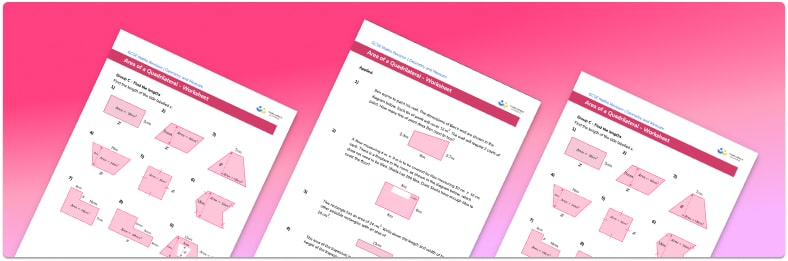• This field is for validation purposes and should be left unchanged.

You can unsubscribe at any time (each email we send will contain an easy way to unsubscribe). To find out more about how we use your data, see our privacy policy.

• Section 1 of the printable area of quadrilaterals worksheet contains 27 skills-based area of quadrilaterals questions, in 2 groups to support differentiation
• Section 2 contains 4 applied area of quadrilaterals questions with a mix of worded problems and deeper problem solving questions
• Answer keys and a mark scheme for all questions are provided
• Questions follow variation theory with plenty of opportunities for students to work independently at their own level
• All questions created by fully qualified expert secondary maths teachers
• Suitable for GCSE maths revision for AQA, OCR and Edexcel exam boards

### Area of quadrilaterals at a glance

Area is the amount of space covered by a 2D shape and is measured in square units (square millimetres, square centimetres and square metres). Many of the area formulae for different types of quadrilaterals (parallelograms, trapezoids, etc) are based on the area of a rectangle.

To work out the area of a rectangle we use the formula length width. The area of the square is calculated in the same way but as the side lengths of a square are all equal, we just need to multiply the side length by itself.

The formula for the area of a parallelogram is the same as the formula for the area of a rectangle. To work out the area of a parallelogram, we use the formula base perpendicular height. As a rhombus is a parallelogram with four equal sides, this formula also works to find the area of a rhombus.

The formula for the area of a trapezium or trapezoid is based on the area of a rectangle. To work out the area of a trapezium, we use the formula (a+b)h divided by 2, where h is the height of the trapezium and a and b are the lengths of the two parallel sides.

In addition to using integers for side lengths, decimals and fractions are also common when calculating area. Students may also be asked to apply multiplication of fractions to the area of quadrilaterals.

Area of a quadrilateral can be extended further to word problems or problems using compound shapes, and to applications involving algebraic expressions, forming and solving equations.

Looking forward, students can then progress to additional geometry worksheets, for example a 3D shapes, faces, vertices and edges worksheet or a surface area worksheetFor more teaching and learning support on Geometry our GCSE maths lessons provide step by step support for all GCSE maths concepts.

## Related worksheets

Angles In Polygons Worksheet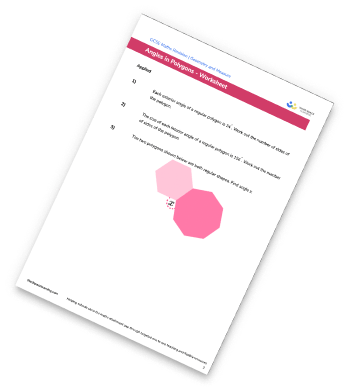Angles in a Triangle Worksheet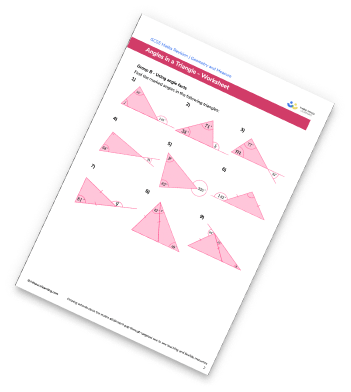Plans And Elevations Worksheet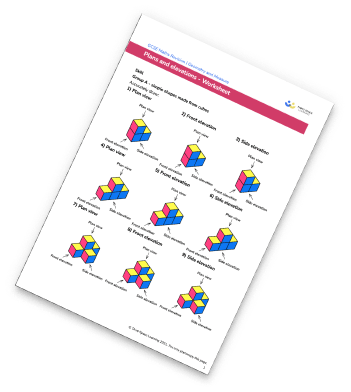Surface Area Worksheets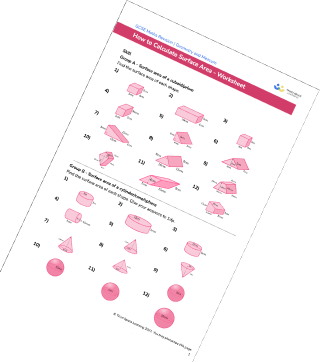## Do you have KS4 students who need more focused attention to succeed at GCSE?There will be students in your class who require individual attention to help them succeed in their maths GCSEs. In a class of 30, it’s not always easy to provide.

Help your students feel confident with exam-style questions and the strategies they’ll need to answer them correctly with our dedicated GCSE maths revision programme.

Lessons are selected to provide support where each student needs it most, and specially-trained GCSE maths tutors adapt the pitch and pace of each lesson. This ensures a personalised revision programme that raises grades and boosts confidence.

Find out more